## Figures index

#### Jaber S. Alzahrani

American Journal of Industrial Engineering. 2019, 6(1), 1-12 doi:10.12691/ajie-6-1-1• Figure 1. Gantt chart of makespan objective []• Figure 2. Optimal makespan distribution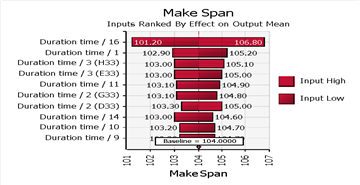• Figure 3. Inputs ranked by the effect on makespan mean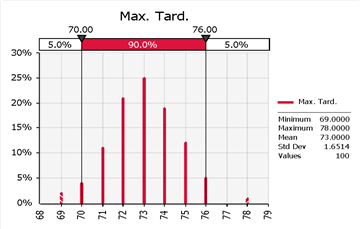• Figure 4. The resulted in maximum tardiness while optimizing the makespan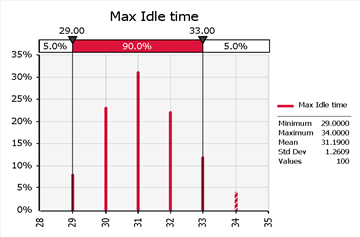• Figure 5. The resulted in maximum machine idle time while optimizing the makespan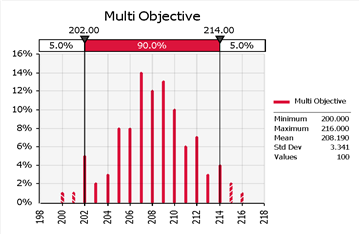• Figure 6. The resulted in multi-objectives (Equal weights) while optimizing the makespan• Figure 7. The resulted in Multi-objectives (double weighted makespan) while optimizing the makespan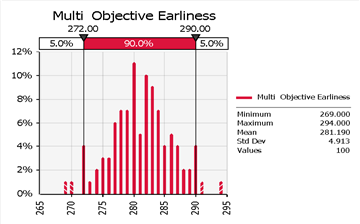• Figure 8. The resulted in Multi-objectives (double weighted maximum job tardiness) while optimizing the makespan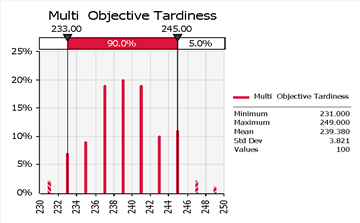• Figure 9. The resulted in Multi-objectives (double weighted maximum machine idle time) while optimizing the makespan• Figure 10. Optimal maximum job tardiness distribution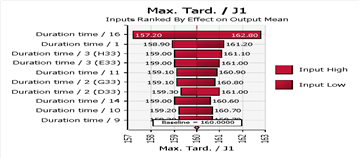• Figure 11. Inputs ranked by the effect on maximum job tardiness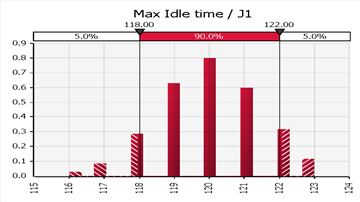• Figure 12. Optimal maximum machine idle time distribution• Figure 13. Inputs ranked by the effect on the maximum machine idle time• Figure 14. Optimal multi-objectives (Equal weights) distribution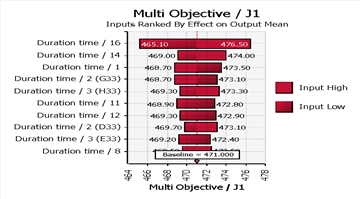• Figure 15. Inputs ranked by the effect on the multi-objectives (Equal weights)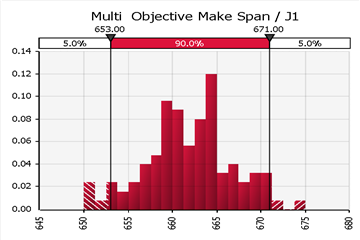• Figure 16. Optimal multi-objective (double weighted makespan) distribution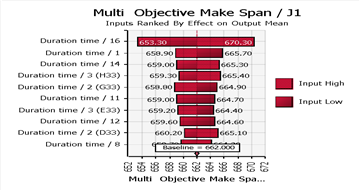• Figure 17. Inputs ranked by the effect on the multi-objectives (double weighted makespan)• Figure 18. Optimal multi-objective (double weighted maximum job tardiness) distribution• Figure 19. Inputs ranked by the effect on the multi-objectives (double weighted maximum job tardiness)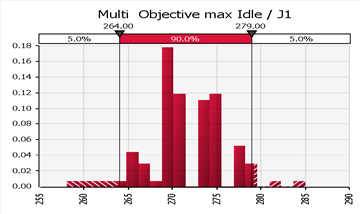• Figure 20. Optimal Multi-objective (double weighted maximum machine idle time) distribution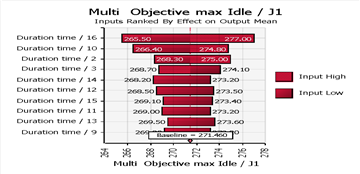• Figure 21. Inputs ranked by the effect on the multi-objectives (double weighted maximum machine idle time)